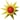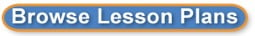Search results for 8th grade math:
 Browse All Lesson Plans Lesson Plan Name Grades 8th grade Math-Intro toTransformations 8 to 8(0 stars, 1 ratings)A week of lesson plans to introduce students to the different Transformations. Interpret the equation 8 to 8(0 stars, 1 ratings)To interpret the equation of line, students will rotate through three stations. Each station will require the students to interpret the equation but using different techniques. Stations: Equation of a line and Slope 8 to 8(0 stars, 1 ratings)This is a stations lesson to check the learner's understanding of graphing lines and slope. The learner will receive immediate feedback at each station to determine if they fully understand the concept.